# 一个例子

• $f(x)=\log(x)$
• $x_{min}=0.99$
• $x_{max}=1.01$
• $x_{min}$$x_{max}$ 之间均匀分布的 1000 个点上，我们观测到 $y_i=f(x_i)+\epsilon_i$，其中 $\epsilon\sim N(0,\sigma^2)$.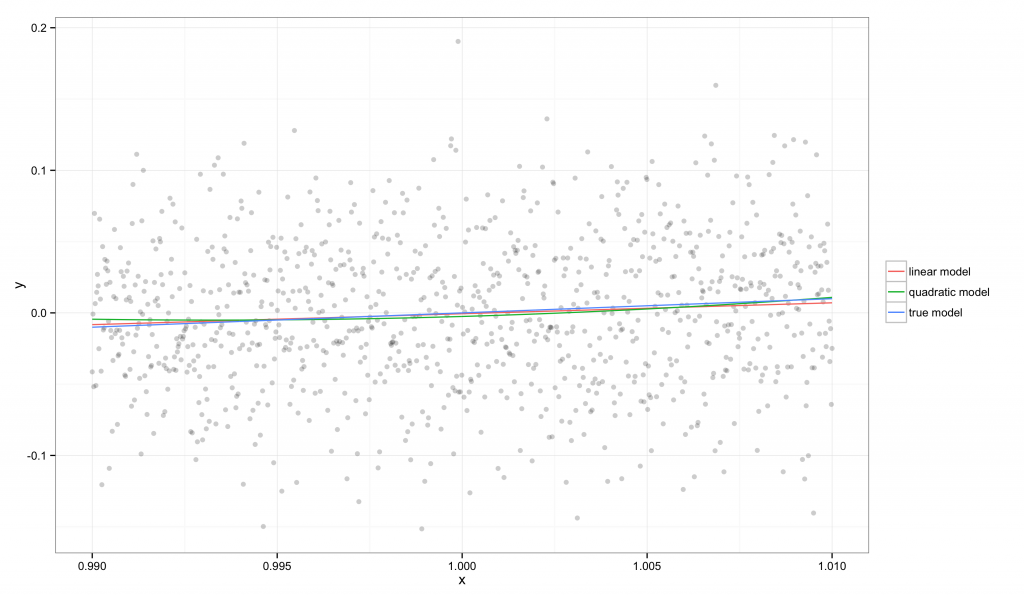在图中，我们可以看到 $f(x)$ 可以被一条直线很好地近似，所以我们的线性和二次回归模型都可以很好地还原真实模型。这是因为 $x_{min}$$x_{max}$ 非常接近，因此真实的函数在这个区域中可以被直线很好地近似，特别是相对于我们观测的噪声水平而言。

$$R^2 = \frac{\text{MSE}_c – \text{MSE}_m}{\text{MSE}_c} = 1 – \frac{\text{MSE}_m}{\text{MSE}_c}$$

$$E^2 = \frac{\text{MSE}_m – \text{MSE}_t}{\text{MSE}_t} = \frac{\text{MSE}_m}{\text{MSE}_t} – 1$$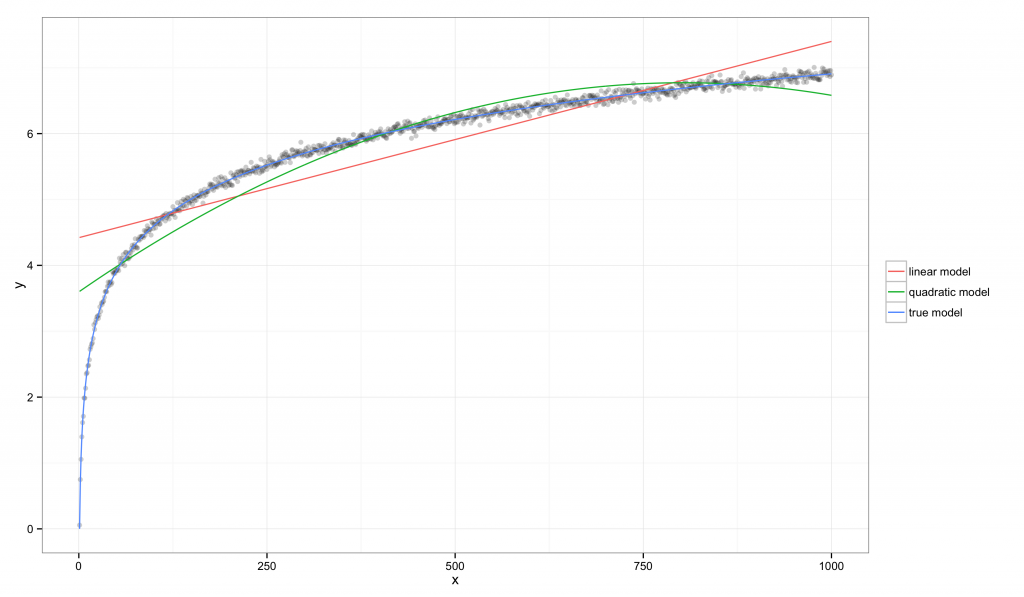在这个例子中，可以通过上图看出线性模型和二次模型都有系统性的偏差，但它们的 $R^2$ 值都有显著的增长：线性模型是 $R^2=0.760$，真实模型是 $R^2=0.997$。相比较而言，线性模型的 $E^2=85.582$，这说明该数据集提供了有力的证据表明线性模型比真实模型要差。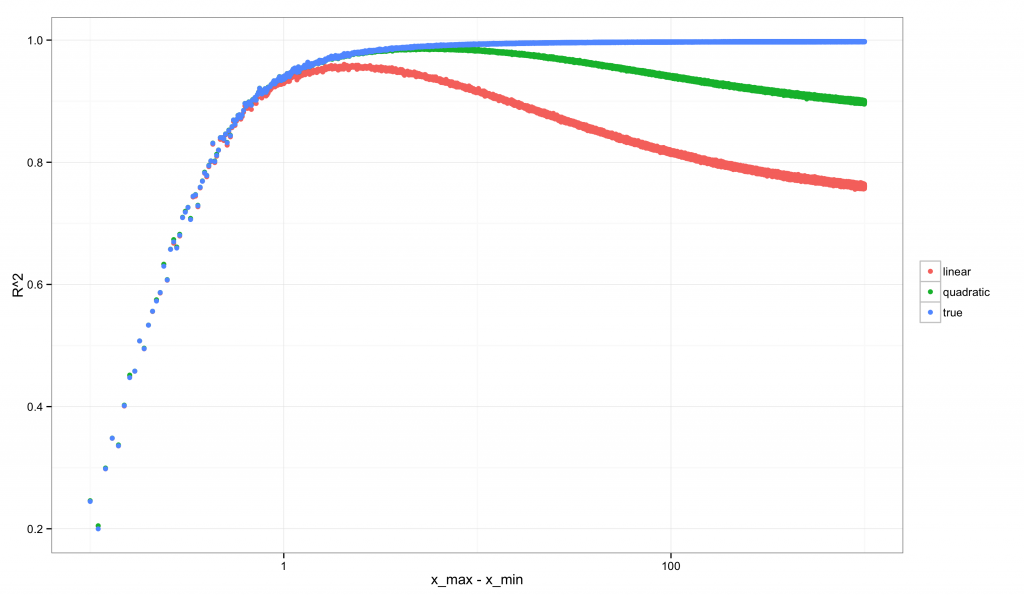其次是 $E^2$ 的：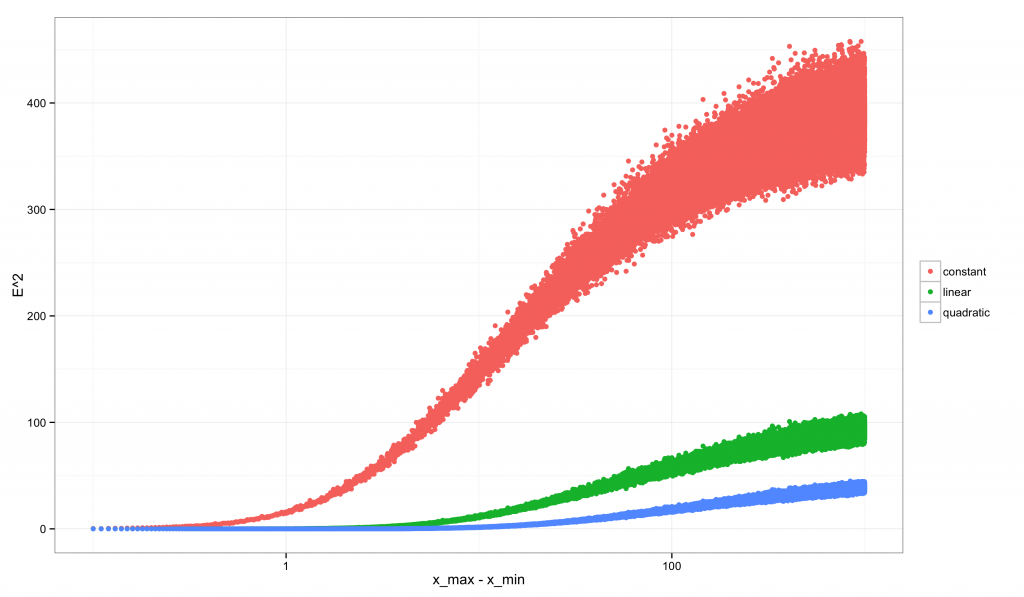注意到 $R^2$ 的图像相对于 $E^2$ 而言是多么的奇特。$E^2$ 始终是递增的：随着间隔的增加我们拥有更多可以区分模型的数据时，我们同时也拥有越来越强的证据表明线性近似不是正确的模型。相反，$R^2$ 一开始非常低（也正是 $E^2$ 很低的时候，因为我们的线性模型略差于真实模型），之后以非单调的模式发生变化：它的峰值位于当数据有足够的变异来排除常数模型，但还不足以排除线性模型的时候。在这个临界点之后，$R^2$ 就开始下降。对于二次模型，$R^2$ 的取值具有类似的非单调性。只有真实的模型展现出单调递增的 $R^2$

# 补记一

$$E^2 = \frac{\text{MSE}_m – \text{MSE}_t}{\text{MSE}_c – \text{MSE}_t}$$

# 补记二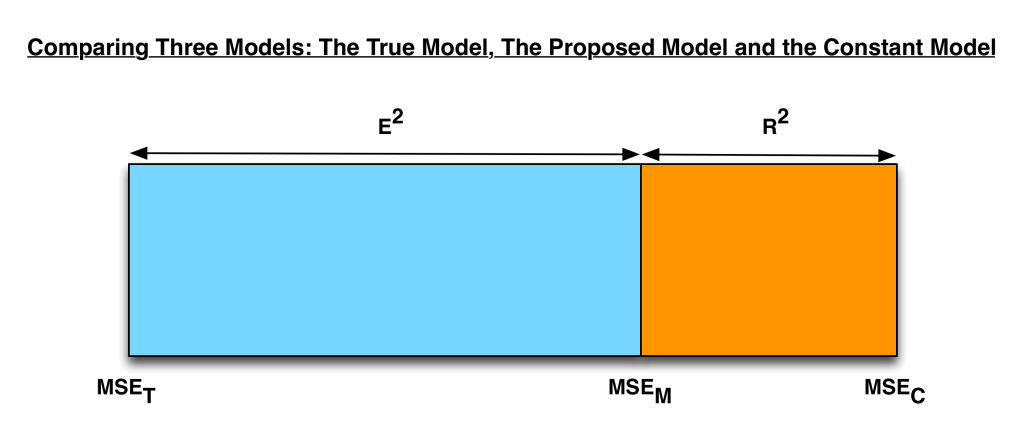在测量备选模型所处的位置时，$R^2$$E^2$ 是互补的关系。

# 补记三

$$R^2 = 1 – \frac{\text{MSE}_m}{\text{MSE}_c} = 1 – \frac{\text{bias}_m^2 + \sigma^2}{\text{bias}_c^2 + \sigma^2}$$

$$E^2 = \frac{\text{MSE}_m – \text{MSE}_t}{\text{MSE}_c – \text{MSE}_t} = \frac{(\text{bias}_m^2 + \sigma^2) – (\text{bias}_t^2 + \sigma^2)} {(\text{bias}_c^2 + \sigma^2) – (\text{bias}_t^2 + \sigma^2)} = \frac{\text{bias}_m^2 – \text{bias}_t^2}{\text{bias}_c^2 – \text{bias}_t^2} = \frac{\text{bias}_m^2 – 0}{\text{bias}_c^2 – 0} = \frac{\text{bias}_m^2}{\text{bias}_c^2}$$